# Fighter

A military fighter flies at an altitude of 10 km. From the ground position, it was aimed at an altitude angle of 23° and 12 seconds later at an altitude angle of 27°. Calculate the speed of the fighter in km/h.

Correct result:

v =  1179.7256 km/h

#### Solution: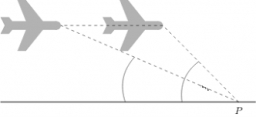We would be pleased if you find an error in the word problem, spelling mistakes, or inaccuracies and send it to us. Thank you!Tips to related online calculators
Do you want to convert length units?
Do you want to convert velocity (speed) units?
Do you want to convert time units like minutes to seconds?
Most natural application of trigonometry and trigonometric functions is a calculation of the triangles. Common and less common calculations of different types of triangles offers our triangle calculator. Word trigonometry comes from Greek and literally means triangle calculation.

#### You need to know the following knowledge to solve this word math problem:

We encourage you to watch this tutorial video on this math problem:

## Next similar math problems:

• Volume per timeHow long does fill take for a pump with a volume flow of 200 l per minute fill a cube-shaped tank up to 75% of its height if the length of the cube edge is 4 m?
• FlowerpotThe block-shaped flowerpot has external dimensions: length 1.25 m, width 10 cm and height 11 cm. The thickness of the boards from which it is made is 0.8 cm. How many liters of soil is needed to fill it 1 cm below the top edge? What surface do we have to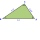The bottom rung of a ladder is 36 inches long and the topmost rung is 24 inches long. If the ladder has 18 rungs, how many inches each other rung is shorter than the rung below it. How many feet of wood was used in making the rungs?
• Coke and coal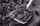20.1 tons of coke is produced from 30 tons of black coal. How much coke is made from 1 kilogram of coal?
• DecibelBy what percentage does the sound intensity increase if the sound intensity level increases by 1 dB?
• RC time constant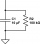You introduced 1 Coulomb worth of electrons into the inner volume of a dielectric material with ϵr=6. 30 minutes later, you found that only 36.79% of the electrons were in the inner volume. Determine the conductivity σ of the dielectric material.
• Double-track line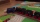A 160 m long passenger train runs on a double-track line in one direction at a constant speed of 54 km/h, and a 240 m long express train in the opposite direction. a) How fast is the express train if passing the passenger train driver for 6 s? b) How long
• Sand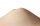How much m ^ 3 of sand can be loaded on a car with a load capacity of 5 t? The sand density is 1600 kg/m ^ 3 .
• Cargo spaceThe cargo space of the truck has the dimensions a = 4.2 m, b = 1.9 m, c = 8.1 dm. Calculate its volume.
• Nautical miles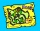How many nautical miles do they sail if the route is shown on a 1:25 000 scale map with a 7.4 cm long line?
• Harmonic oscillator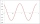Calculate the total energy of a body performing a harmonic oscillating motion if its mass is 200 g, the amplitude of the deflection is 2 cm and the frequency is 5 Hz. Round the result to 3 decimal places.
• Concrete columnThe concrete column of the highway bridge has the shape of a block with dimensions of 1m x 0.8m x 25m. It should be lifted by crane to a height of 20m. What is the power of his engine if the lifting takes 2 minutes?
• Isosceles trapezoidFind the area of an isosceles trapezoid with bases of 8cm and 72mm. The height of the trapezoid is equal to three-quarters of the longer base.
• Two vases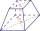Michaela has two vases in her collection. The first vase has the shape of a cone with a base diameter d = 20 cm; the second vase has the shape of a truncated cone with the diameter of the lower base d1 = 25 cm and with the diameter of the upper base d2 =
• AquariumHow many liters of water can fit in an aquarium measuring 30, 15 and 20 cm?
• The bodyThe body has dimensions of 2m 2dm and 10 cm. It weighs 28 kg. What is its density?
• A section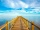A section of the boardwalk is made of 15 boards. Each board is 9 1/4 inches wide. The total width of the boardwalk is 144 inches. The spacing between each board is equal. What is the width of the spacing between the boards?